# RD Sharma Solutions for Class 11 Maths Exercise 22.1 Chapter 22 - Brief review of Cartesian System of Rectangular Coordinates

Here, in this exercise, we shall discuss concepts related to cartesian coordinates of a point, the distance between two points and finding the area of a triangle. Students who aim to secure good marks in the board exams are advised to refer to RD Sharma Class 11 Maths Solutions. The solutions are formulated by our expert tutors in a step by step manner to help students understand the concepts clearly. Students are advised to practice regularly on a daily basis to come out with flying colours in their board examination. The pdf of RD Sharma Solutions are provided in the links given below, which can be downloaded easily and can be used for future reference as well.

## Download the pdf of RD Sharma Solutions for Class 11 Maths Exercise 22.1 Chapter 22 – Brief review of Cartesian System of Rectangular Coordinates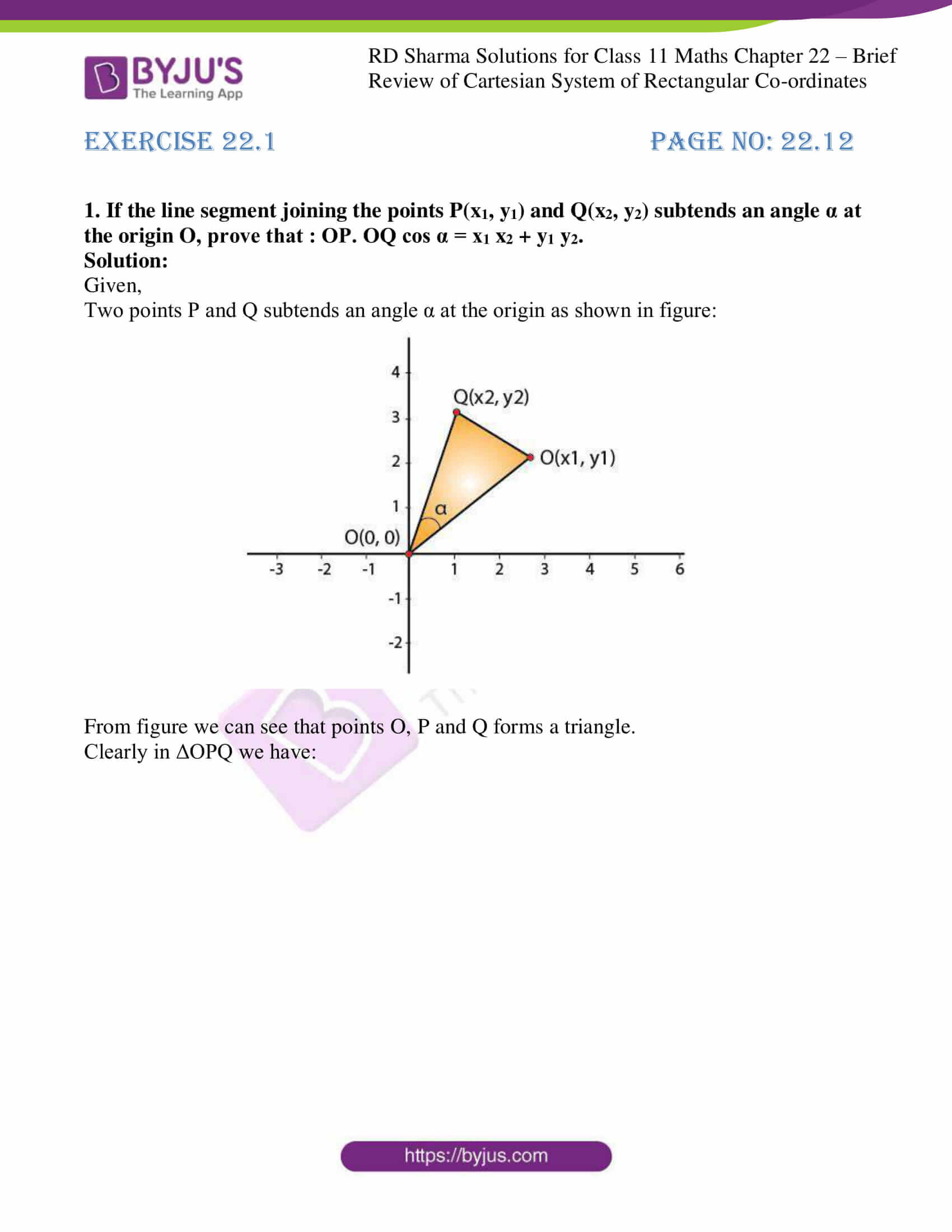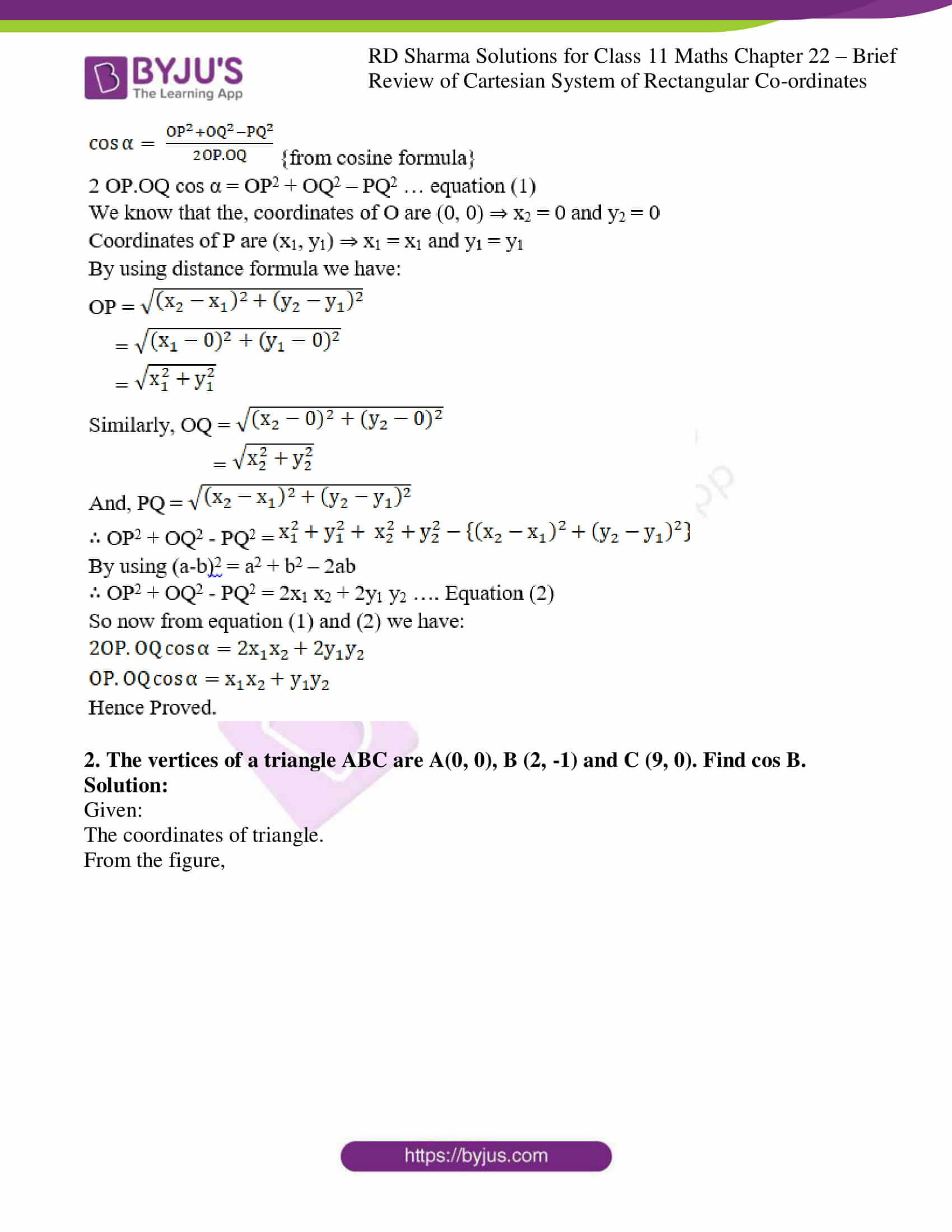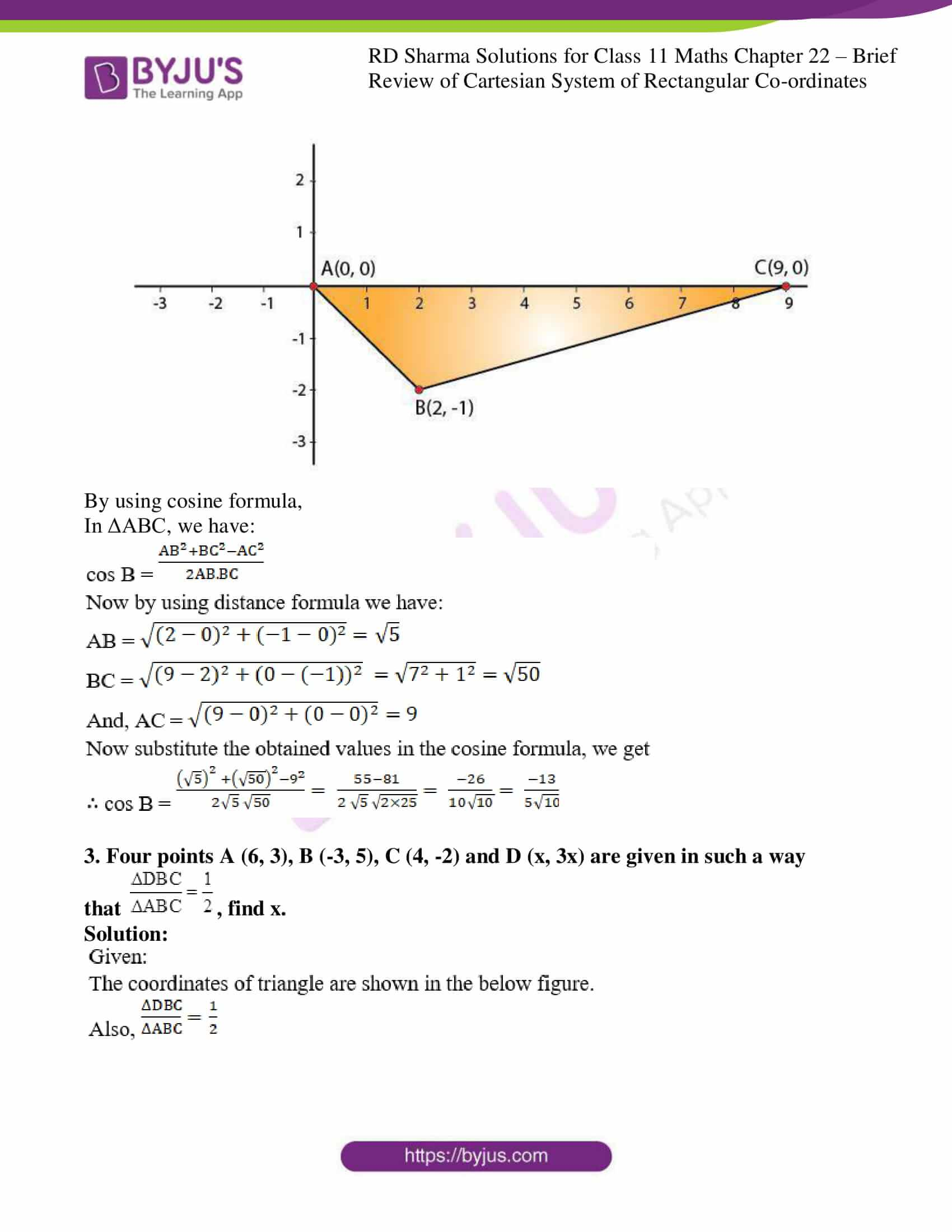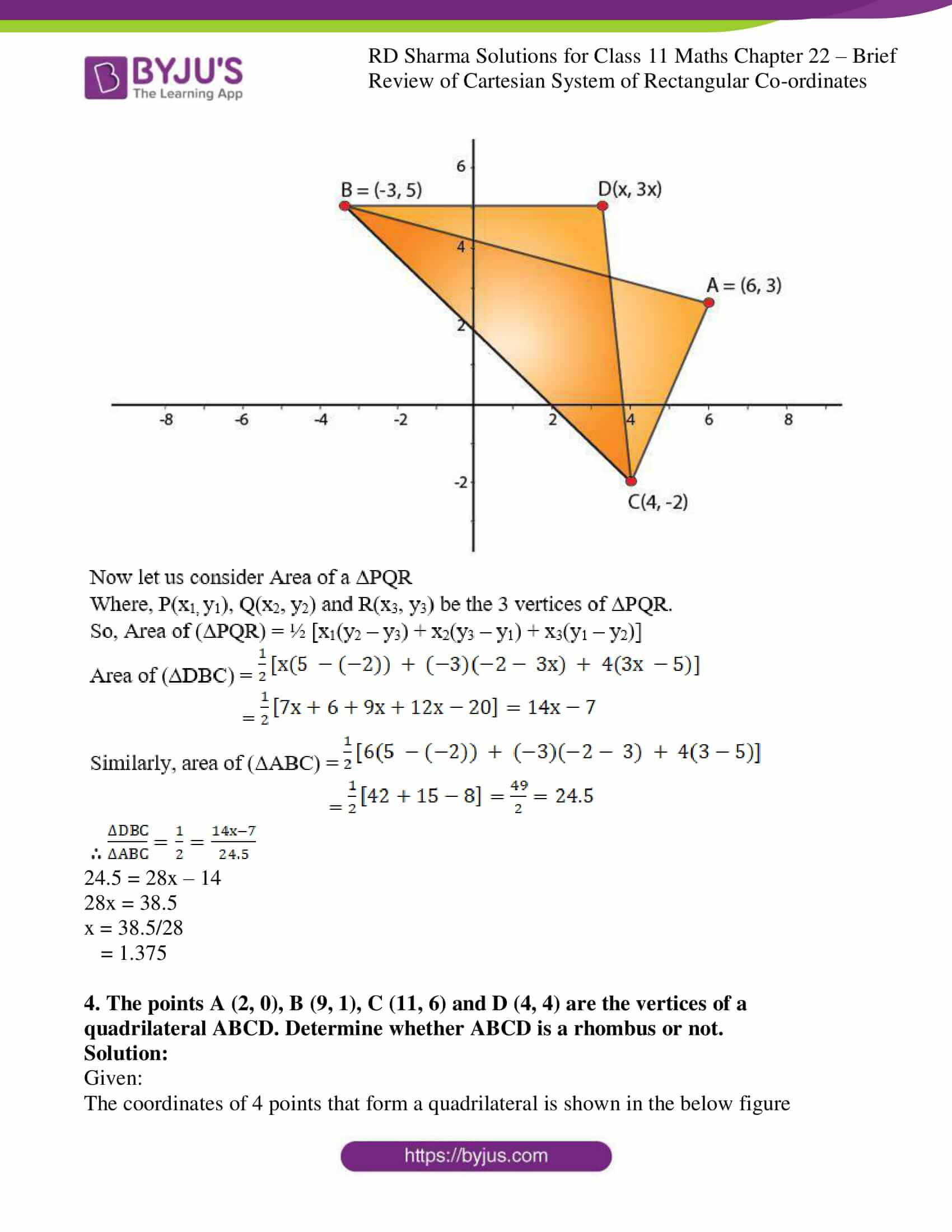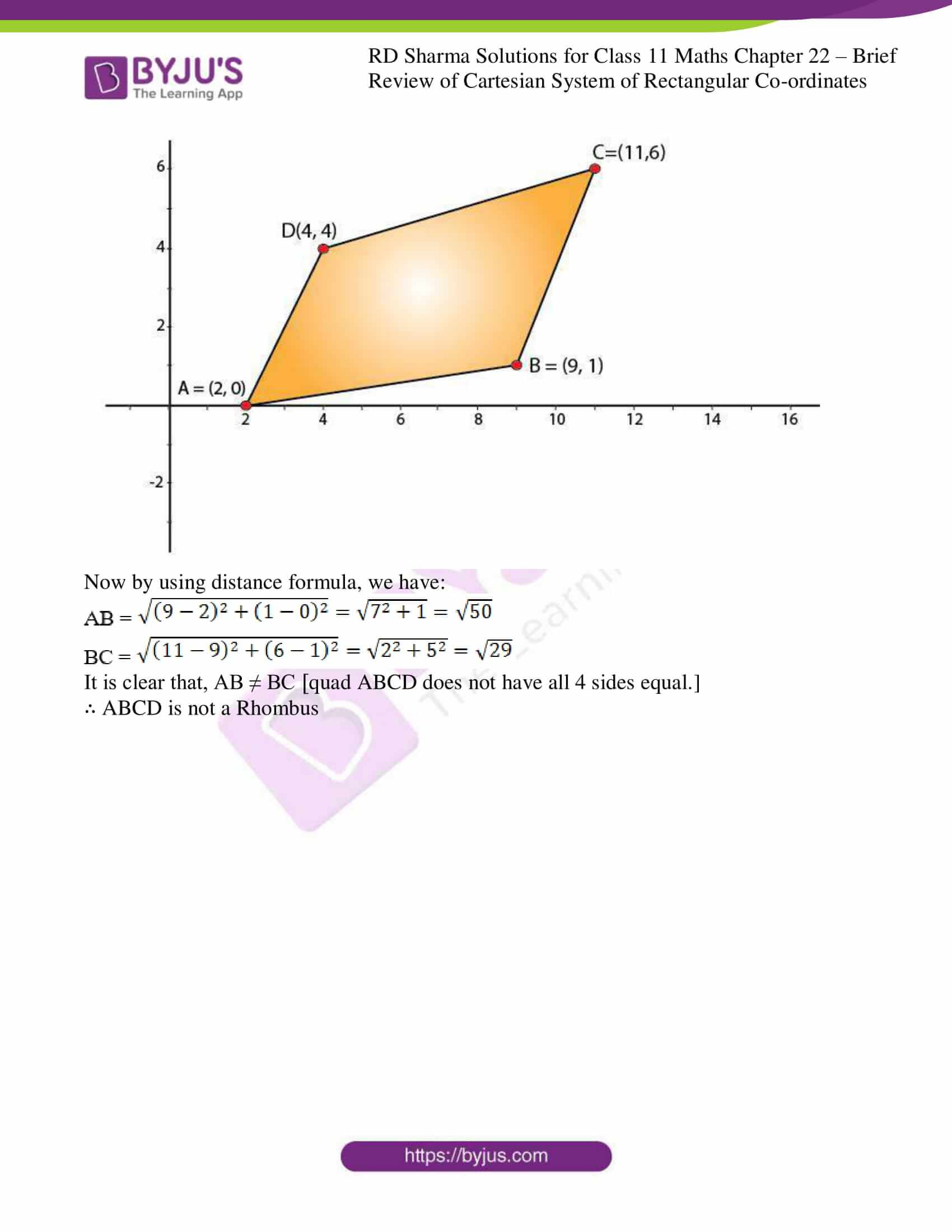### Also, access other exercises of RD Sharma Solutions for Class 11 Maths Chapter 22 – Brief review of Cartesian System of Rectangular Coordinates

Exercise 22.2 Solutions

Exercise 22.3 Solutions

### Access answers to RD Sharma Solutions for Class 11 Maths Exercise 22.1 Chapter 22 – Brief review of Cartesian System of Rectangular Coordinates

1. If the line segment joining the points P(x1, y1) and Q(x2, y2) subtends an angle α at the origin O, prove that : OP. OQ cos α = x1 x2 + y1 y2.

Solution:

Given,

Two points P and Q subtends an angle α at the origin as shown in figure: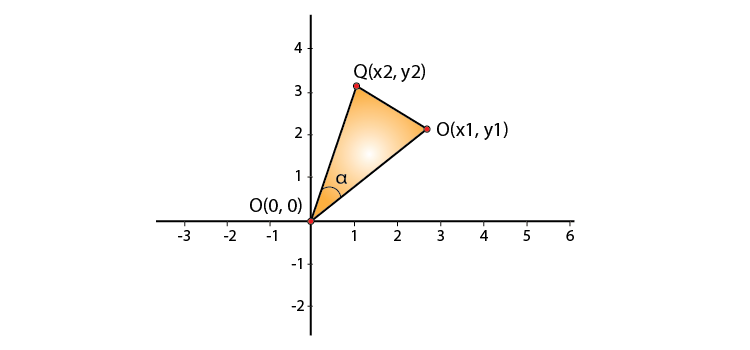From figure we can see that points O, P and Q forms a triangle.

Clearly in ΔOPQ we have: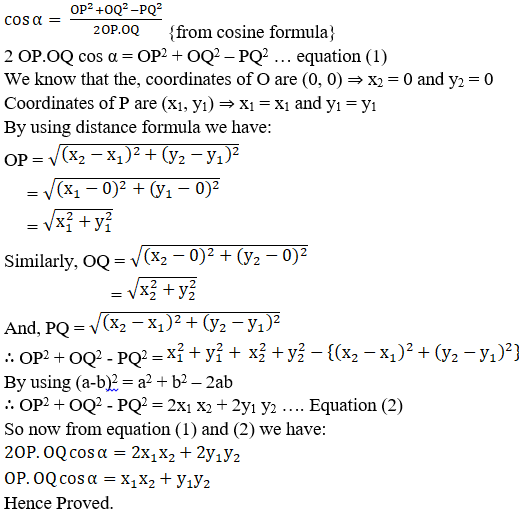2. The vertices of a triangle ABC are A(0, 0), B (2, -1) and C (9, 0). Find cos B.

Solution:

Given:

The coordinates of triangle.

From the figure,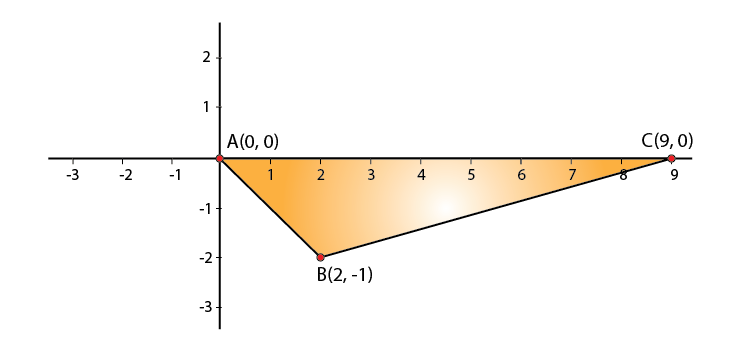By using cosine formula,

In ΔABC, we have: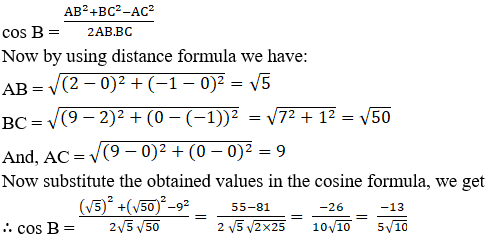3. Four points A (6, 3), B (-3, 5), C (4, -2) and D (x, 3x) are given in such a way that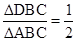, find x.

Solution: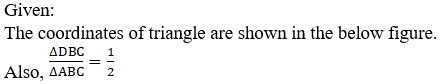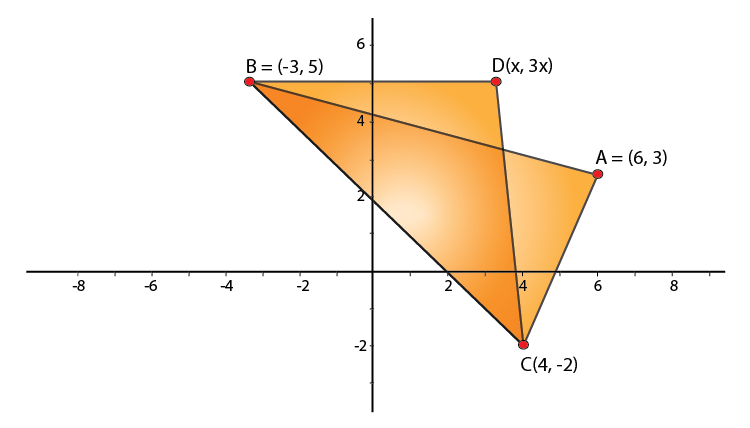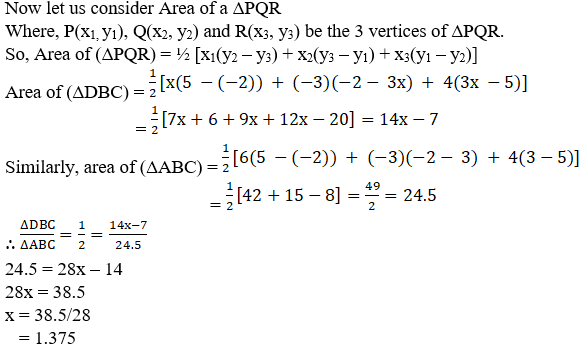24.5 = 28x – 14

28x = 38.5

x = 38.5/28

= 1.375

4. The points A (2, 0), B (9, 1), C (11, 6) and D (4, 4) are the vertices of a quadrilateral ABCD. Determine whether ABCD is a rhombus or not.

Solution:

Given:

The coordinates of 4 points that form a quadrilateral is shown in the below figure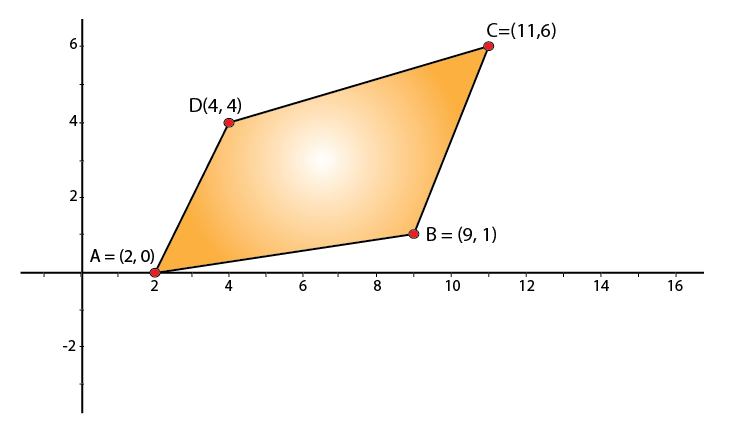Now by using distance formula, we have: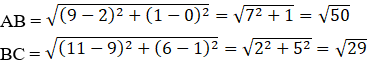It is clear that, AB ≠ BC [quad ABCD does not have all 4 sides equal.]

∴ ABCD is not a Rhombus